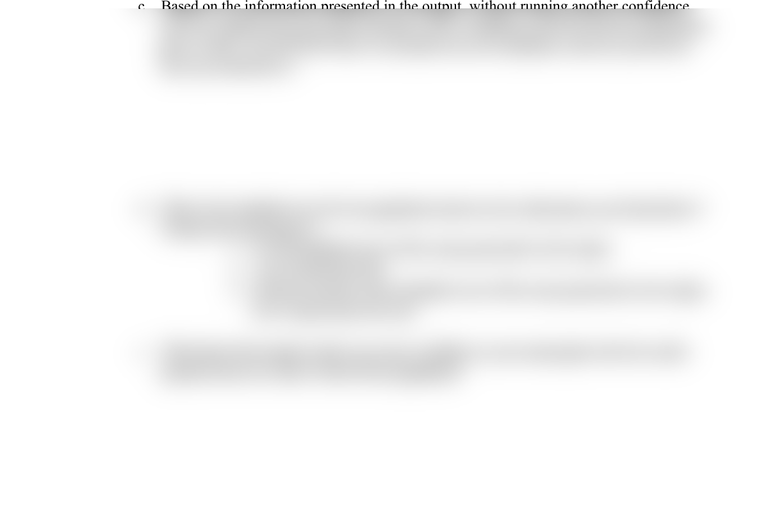Textbook Notes (270,000)
US (100,000)
LSU (20,000)
SOCL (1,000)
SOCL 2001 (1,000)
All (1,000)
Chapter

LA 3

Department
Sociology
Course Code
SOCL 2001
Professor
All

This preview shows half of the first page. to view the full 1 pages of the document.Statistics Lab 2201 Name: ____________________________
Lab Assignment #3
Due: October 22, 2008
Directions: Using the GSS96small data set, answer the following questions.
1. Run an estimate of the standard error of the mean for the variable age.
a. What is the estimate of the standard error of the mean? ____________________
b. Why do we refer to this as an estimate rather than the true standard error?
2. Run a confidence interval for the variable occupational prestige (prestg80).
a. What is the estimate of the standard error of the mean? ____________________
b. Indicate where 95% of the cases will fall. From ______________ to ______________.
c. Based on the information presented in the output, without running another confidence
interval, explain how you could calculate a 99% confidence interval with the information
given. (Note: You DO NOT have to calculate the new confidence interval, just tell me
how you would do it.)
d. What is the standard error for the population based on the information you found above?
1. It is the standard error of the mean presented in the output.
2. I can’t determine this.
3. My best estimate is the standard error of the mean presented in the output,
but I cannot know for sure.